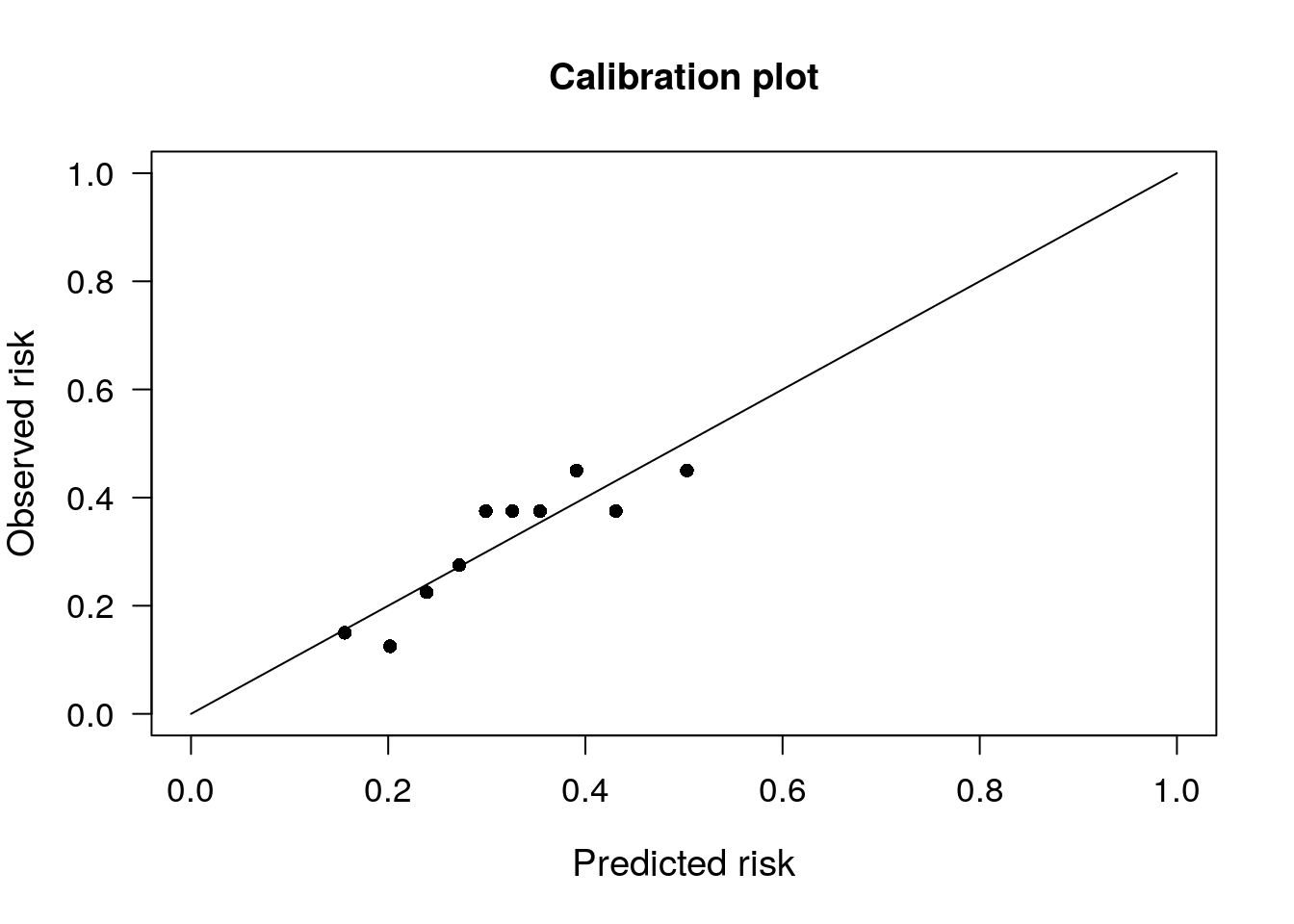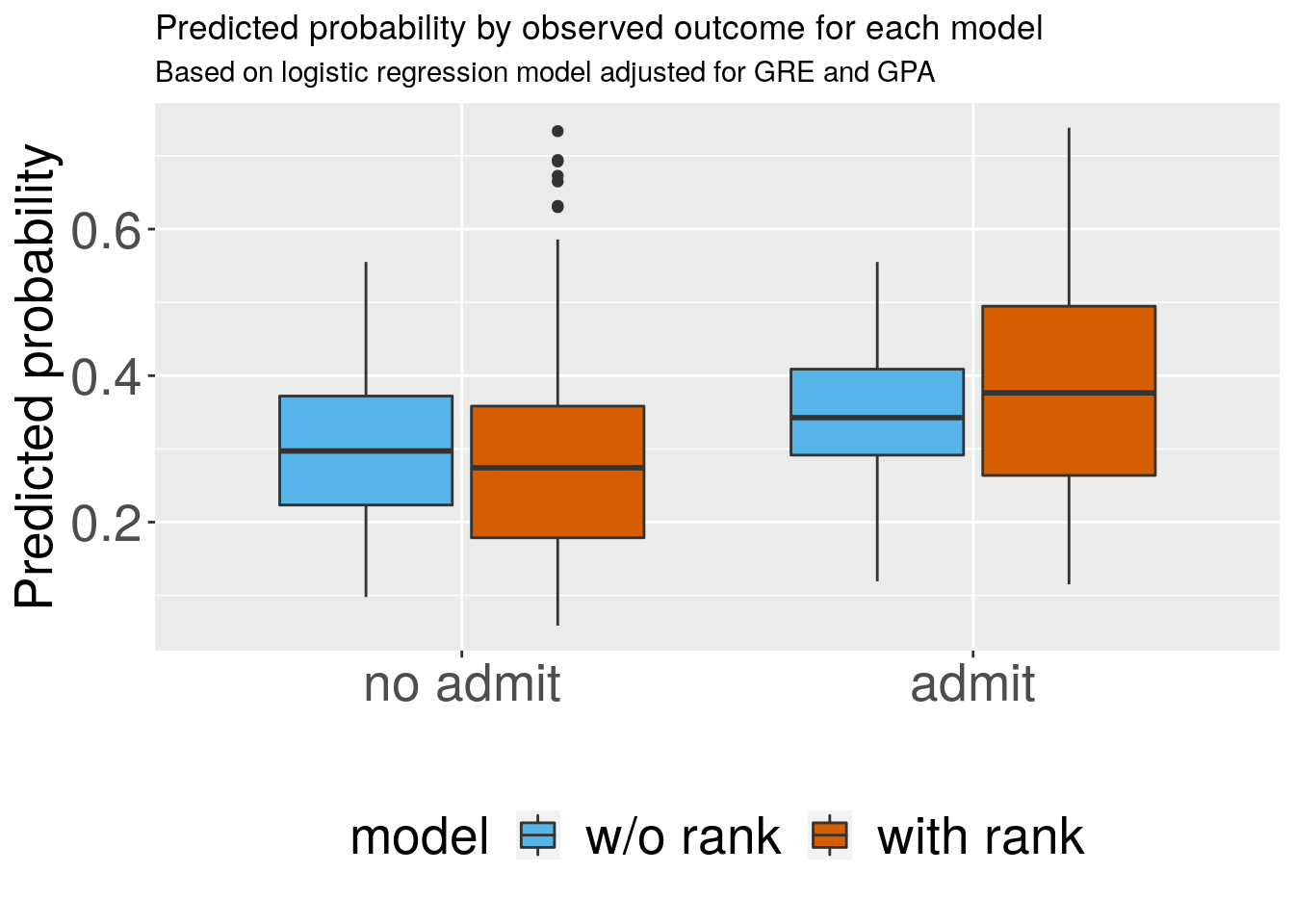# Calibration, Net Re-Classification Index and Goodness-of-Fit Test for Logistic Regression

This post shows how to calculate the Net Re-Classification Index and Goodness-of-Fit Test (Hosmer-Lemeshow Test) for logistic regression models. In addition, we show how to plot the calibration curves.

#  load packages ----------------------------------------------------------

if (!requireNamespace("pacman", quietly = TRUE)) {
install.packages("pacman")
}

# packages for ggplot2 themes

# colors and themes -------------------------------------------------------

# color blind palette
cbbPalette <- c("#999999", "#E69F00", "#56B4E9", "#009E73", "#F0E442", "#0072B2", "#D55E00", "#CC79A7")

# my theme defaults
gg_sy <- theme(legend.position = "bottom",
axis.text = element_text(size = 20),
axis.title = element_text(size = 20),
legend.text = element_text(size = 20),
legend.title = element_text(size = 20))

#  load data --------------------------------------------------------------

## view the first few rows of the data
head(mydata)
##   admit gre  gpa rank
## 1     0 380 3.61    3
## 2     1 660 3.67    3
## 3     1 800 4.00    1
## 4     1 640 3.19    4
## 5     0 520 2.93    4
## 6     1 760 3.00    2
mydata$rank <- factor(mydata$rank)
mydata$admitf <- factor(mydata$admit, levels = c(0,1), labels = c("no admit", "admit"))

# without rank
m1 <- glm(admit ~ gre + gpa, data = mydata, family = "binomial")
preds_m1 <- predict(m1, type = "response") # predicted probs from model 1

# with rank
m2 <- glm(admit ~ gre + gpa + rank, data = mydata, family = "binomial")
preds_m2 <- predict(m2, type = "response") # predicted probs from model 2

# Calibration plots and Hosmer Lemeshow test ------------------------------

# In this example, we see that the $Chi_square value is smaller for m2, compared to m1 # The null hypothesis is that we have a good fit. So the larger the p-value, the smaller the # Chi_square statistic, the better the fit. So we can say that rank has added value to the model # Calibration plot for m1 PredictABEL::plotCalibration(data = as.matrix(mydata$admit), # observed response
cOutcome = 1, # the category corresponding to outcome of interest
predRisk = preds_m1, # predicted probs
groups = 10 # number of groups to bin the predicted probabilities into, usually 10 is default
)
## $Table_HLtest ## total meanpred meanobs predicted observed ## [0.0978,0.184) 40 0.156 0.150 6.25 6 ## [0.1839,0.224) 40 0.202 0.125 8.09 5 ## [0.2241,0.254) 40 0.239 0.225 9.56 9 ## [0.2536,0.289) 40 0.272 0.275 10.89 11 ## [0.2894,0.311) 40 0.299 0.375 11.97 15 ## [0.3110,0.341) 40 0.326 0.375 13.04 15 ## [0.3406,0.370) 40 0.354 0.375 14.16 15 ## [0.3702,0.408) 40 0.391 0.450 15.64 18 ## [0.4079,0.461) 40 0.431 0.375 17.26 15 ## [0.4614,0.555] 40 0.503 0.450 20.13 18 ## ##$Chi_square
##  4.706
##
## $df ##  8 ## ##$p_value
##  0.7885
# Calibration plot for m2
PredictABEL::plotCalibration(data = as.matrix(mydata$admit), # observed response cOutcome = 1, # the category corresponding to outcome of interest predRisk = preds_m1, # predicted probs groups = 10 # number of groups to bin the predicted probabilities into, usually 10 is default, so you will see 10 categories and 10 points on the calibration plot )##$Table_HLtest
##                total meanpred meanobs predicted observed
## [0.0978,0.184)    40    0.156   0.150      6.25        6
## [0.1839,0.224)    40    0.202   0.125      8.09        5
## [0.2241,0.254)    40    0.239   0.225      9.56        9
## [0.2536,0.289)    40    0.272   0.275     10.89       11
## [0.2894,0.311)    40    0.299   0.375     11.97       15
## [0.3110,0.341)    40    0.326   0.375     13.04       15
## [0.3406,0.370)    40    0.354   0.375     14.16       15
## [0.3702,0.408)    40    0.391   0.450     15.64       18
## [0.4079,0.461)    40    0.431   0.375     17.26       15
## [0.4614,0.555]    40    0.503   0.450     20.13       18
##
## $Chi_square ##  4.706 ## ##$df
##  8
##
## $p_value ##  0.7885 # Boxplots for discrimination --------------------------------------------- df1 <- data.frame(admit = mydata$admitf,
model = "w/o rank",
predicted = preds_m1,
stringsAsFactors = FALSE)

df2 <- data.frame(admit = mydata$admitf, model = "with rank", predicted = preds_m2, stringsAsFactors = FALSE) dfplot <- rbind(df1, df2) dfplot$model <- factor(dfplot$model) ggplot(dfplot, aes(x = admit, y = predicted, fill = model)) + geom_boxplot() + scale_fill_manual(values=cbbPalette[c(3,7)], guide=guide_legend(ncol = 2)) + labs(x="", y="Predicted probability", title="Predicted probability by observed outcome for each model", subtitle="Based on logistic regression model adjusted for GRE and GPA", caption="") + theme_ipsum_rc() + theme(legend.position = "bottom", axis.text = element_text(size = 20), axis.title = element_text(size = 20), legend.text = element_text(size = 20), legend.title = element_text(size = 20))# Net reclassification index ---------------------------------------------- #' Re-classfication tables quantify the model improvement of one model over #' another by counting the number of individuals who move to another risk #' category or remain in the same risk category as a result of updating the risk #' model. Any _upward_ movement in categories for event subjects (i.e. those #' with admit=1) implies improved classification, and any _downward_ movement #' indicates worse reclassification. The interpretation is opposite for people #' who do not develop events (admit = 0). Smaller p-values indicate better #' reclassification accuracy. #' PredictABEL::reclassification(data = as.matrix(mydata$admit),
cOutcome = 1,
predrisk1 = preds_m1, # original model
predrisk2 = preds_m2, # updated model
cutoff = seq(0, 1, by = 0.20))
##  _________________________________________
##
##      Reclassification table
##  _________________________________________
##
##  Outcome: absent
##
##              Updated Model
## Initial Model [0,0.2) [0.2,0.4) [0.4,0.6) [0.6,0.8) [0.8,1]
##     [0,0.2)        31        16         0         0       0
##     [0.2,0.4)      52        99        24         0       0
##     [0.4,0.6)       0        26        18         7       0
##     [0.6,0.8)       0         0         0         0       0
##     [0.8,1]         0         0         0         0       0
##              Updated Model
## Initial Model  % reclassified
##     [0,0.2)                34
##     [0.2,0.4)              43
##     [0.4,0.6)              65
##     [0.6,0.8)             NaN
##     [0.8,1]               NaN
##
##
##  Outcome: present
##
##              Updated Model
## Initial Model [0,0.2) [0.2,0.4) [0.4,0.6) [0.6,0.8) [0.8,1]
##     [0,0.2)         7         2         0         0       0
##     [0.2,0.4)      11        40        28         0       0
##     [0.4,0.6)       0        11        15        13       0
##     [0.6,0.8)       0         0         0         0       0
##     [0.8,1]         0         0         0         0       0
##              Updated Model
## Initial Model  % reclassified
##     [0,0.2)                22
##     [0.2,0.4)              49
##     [0.4,0.6)              62
##     [0.6,0.8)             NaN
##     [0.8,1]               NaN
##
##
##  Combined Data
##
##              Updated Model
## Initial Model [0,0.2) [0.2,0.4) [0.4,0.6) [0.6,0.8) [0.8,1]
##     [0,0.2)        38        18         0         0       0
##     [0.2,0.4)      63       139        52         0       0
##     [0.4,0.6)       0        37        33        20       0
##     [0.6,0.8)       0         0         0         0       0
##     [0.8,1]         0         0         0         0       0
##              Updated Model
## Initial Model  % reclassified
##     [0,0.2)                32
##     [0.2,0.4)              45
##     [0.4,0.6)              63
##     [0.6,0.8)             NaN
##     [0.8,1]               NaN
##  _________________________________________
##
##  NRI(Categorical) [95% CI]: 0.2789 [ 0.1343 - 0.4235 ] ; p-value: 0.00016
##  NRI(Continuous) [95% CI]: 0.4543 [ 0.2541 - 0.6545 ] ; p-value: 1e-05
##  IDI [95% CI]: 0.0546 [ 0.0309 - 0.0783 ] ; p-value: 1e-05